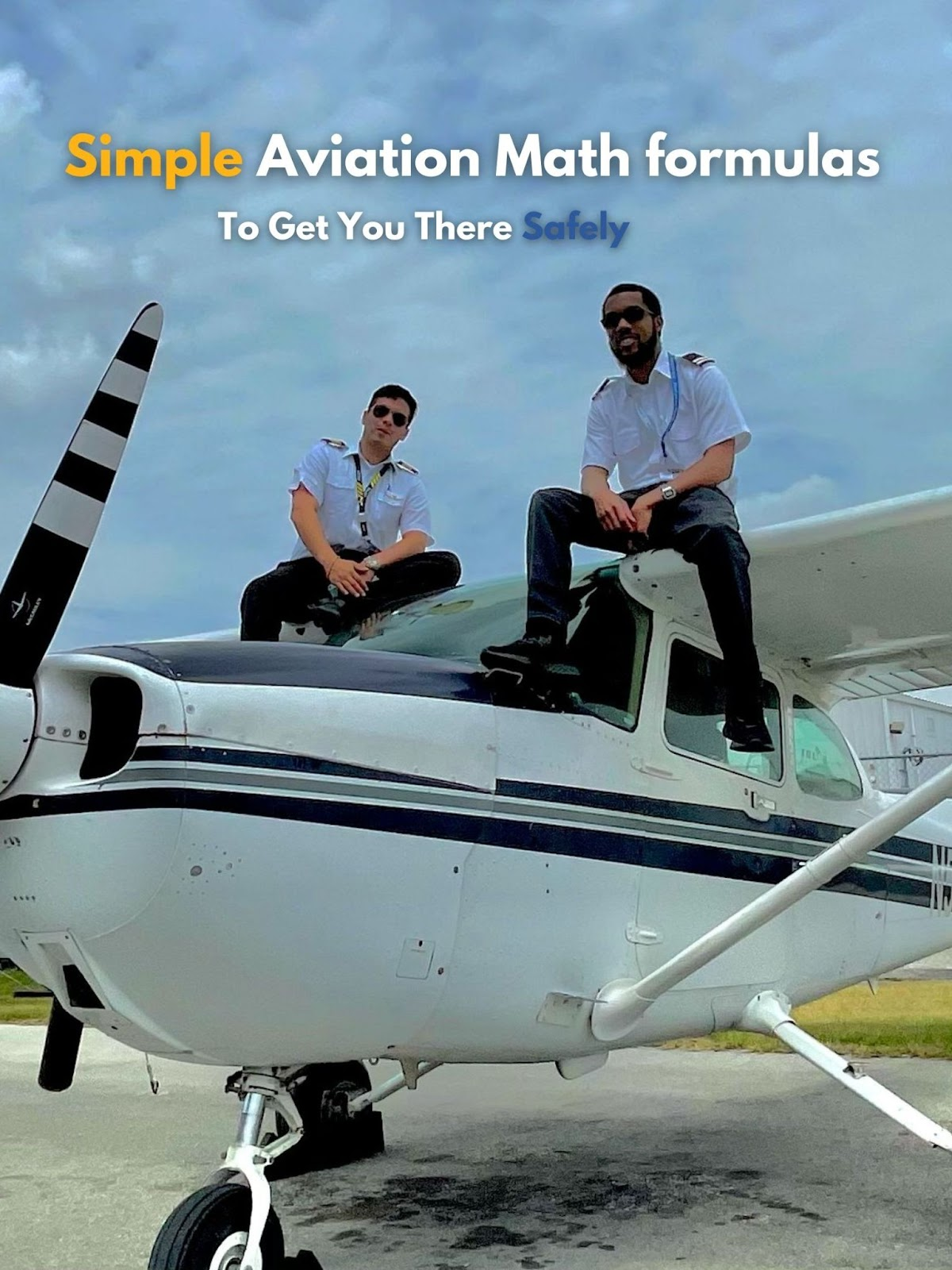Pilots love to fly, but aren’t always fans of calculations. When you’re in the cockpit simple aviation mental math will help you “stay ahead of the plane.” It reduces workload and will make you a better, safer pilot. Sometimes flying involves a lot of numbers, especially preflight planning for an instrument flight or when briefing an approach. Most of the math that may need to be done is simple but often remembering the right arithmetic or formulas can be confusing and complex.

Fortunately there are simpler formulas that can be done mentally or quickly on a calculator that can make your flight more precise. Here are 7 simple formulas to make your flight more precise:

1. Calculating Maneuvering Speed ( Va ) is crucial because it is the speed at which full deflection of any flight control surface may cause damage to the structure of the aircraft. It is also recommended that if turbulence is encountered you should be below Va. The Pilot Operating Handbook (POH) of most small aircraft has a recommended maneuvering speed when at max gross weight. It is important to note however, that at different gross weights Va changes.

A simple formula to determine Va based on current gross weight is Square root of (Current Takeoff weight / Max gross takeoff weight) X published Va. Cessna 172 example: square root of (2100 / 2300) * 97 KIAS = 92.7 rounded up to 93KIAS.

1. Calculating Visual Descent Point (VDP) - A VDP is a point on the final approach course on a non-precision straight in approach from which a stable visual descent from the minimum descent altitude (MDA) can be made. It helps by making approach planning more precise and gives the pilot a better chance at making a stable descent to the runway instead of diving down.

To calculate a VDP: Height Above Touchdown (HAT) / 300 (for a 3 degree glide slope) example: 450 / 300 = 1.5 which means a VDP can be used 1.5 miles from the runway. You can read more in depth about VDPs here.

1. Converting climb gradient from feet per nautical mile to feet per minute (FPM) - On obstacle departure procedures (ODPs) and standard instrument departures (SIDs) climb gradients in feet per nautical mile are often given to guarantee obstacle clearance.

The terminal procedures publication (TPP) has a table that can be used to convert to feet per minute but this simple formula can also be used. Ground speed / 60 x Feet per nautical mile. Example: 70/60 x 200 ft. Per nautical mile = 233.33 feet per minute

1. How far out to start descending (using a standard 3 degree glide path) - Descent planning helps to make a flight more precise and efficient. There are two simple formulas that can be used.

The first is: Multiplying your height above field elevation by 3. This would give the distance to start descending at 500 FPM. Example: 6000 ft above field x 3 = 18,000 Then drop the 3 zeros to get 18. Which means you can start descending 18 miles out.

Another formula to use is: Altitude to lose / 300 (3 degree glide) example: 6000 / 300 = 20. Which would mean start descending 20 miles out for a 3 degree glide path.

1. Rate of descent to track a glide slope (3 degree) - When flying a precision approach the glide slope can simply be followed to track a stable glide path to the runway. However, staying ahead of the airplane is always ideal and calculating your rate of descent to track the glide slope may provide more situational awareness.

To calculate use: (groundspeed / 60) x 300. Example: (90/60) x 300 = 450 feet per minute. Note that 300 is used because a 3 degree glide slope is a descent of 300 feet per nautical mile. If the glide slope angle is 3.2 degrees then 320 can be used.

1. Descent rate to use from the final approach fix (FAF) to a point before the runway on non-precision approaches - During non-precision approaches sometimes more planning is needed due to less guidance. Determining a descent rate to use from the FAF to a point before the runway can help to make the approach more stable and add situational awareness.

The formula for this is: (altitude to lose/distance) x (groundspeed/60) Example:

• Ground speed of 90 knots
• Minimum descent altitude (MDA) of 500 feet MSL
• You are at 2000 feet MSL at the FAF
• The FAF is 5 miles from the runway
• You want to be at the MDA 1 nautical mile before the runway

(1500 / 4) x (90/60) = 562.5 feet per minute descent.

1. Calculate the climb gradient required to climb over an obstacle - At some airports, explicit obstacle departure procedures are not published. Instead there may be information published about what obstacles are nearby, how far they are and the height of the largest obstacles. In these cases it may be wise to calculate the climb gradient required to clear those obstacles, especially if going IFR.

This formula can be used: Obstacle height / Distance from airport. Example: 1000 foot obstacle, 4NM from airport. 1000 / 4 = 250 feet per nautical mile. The 250 ft. Per NM can then be converted to a FPM climb rate.

A proper and detailed planned flight can offer greater situational awareness, precision, efficiency and overall safety. This is especially important during IFR or in IMC. What are some simple math formulas that you have used when flying? Comment below!